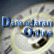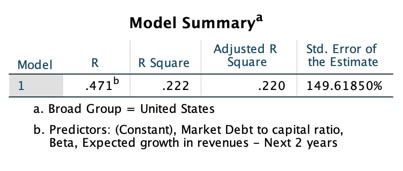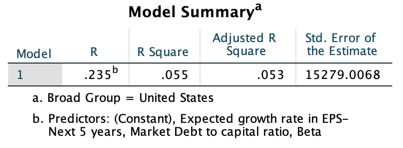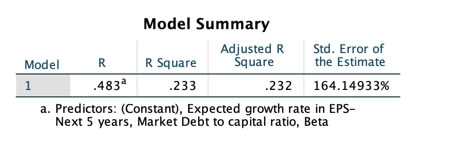##Dividend Regressions: January 2019

Variables used in the regression

1. Dividend Yield = Dividends per share in most recent year/ Current Stock Price
2. Dividend Payout Ratio = Dividends / Net Income
3. Beta: Regression or Bottom up beta
4. Expected Growth in EPS over next 5 years = Consensus analyst estimate (or your own) of expected growth in EPS . If you don't have an analyst estimate, use your own estimate of expected growth.
5. Market Debt to Capital = Debt/ (Debt + Market Value of Equity): If you have market value for debt, use it. If not, use book value of debt and market value of equity.

US Regression: Dividend Yield### US Regression Output

Dividend Yield = .0318 - .0001 Beta - 0.03 Expected Growth +.025 Market Debt to Capital Ratio

T statistic on intercept = 26.09

T statistic on beta = 10.16

T statistic on expected growth = 7.12

T statistic on debt to capital ratio = 13.24

US Regression: Dividend Payout### Regression Output

Dividend Payout = 1.53 -.540 Beta - 1.829 Expected Growth +.28 Market Debt to Capital Ratio

T statistic on intercept = 12.89

T statistic on beta = 5.26

T statistic on expected growth = 1.50

T statistic on debt to capital ratio = 4.42

Global Regression: Dividend Yield### Regression Output

Dividend Yield = 0.028 -.0006 Beta - 0.053 Expected Growth +.032 Market Debt to Capital Ratio

T statistic on intercept = 41.47

T statistic on beta = 15.83

T statistic on expected growth = 3.28

T statistic on debt to capital ratio = 26.82

Global Regression: Dividend Payout### Regression Output

Dividend Payout = .81 -.171 Beta -0.59 Expected Growth +.16 Market Debt to Capital Ratio

T statistic on intercept = 20.69

T statistic on beta = 5.83

T statistic on expected growth = 10.12

T statistic on debt to capital ratio = 14.31

• How do I use this regression?

Assume that you want to estimate the dividend payout ratio for a firm with the following characteristics, using the US regression:

Regression beta = 1.20

Expected Growth in EPS over next 5 years = 10%

Market Debt to Capital = 30%

• Predicted Values
• Expected Dividend Yield = .0318 - .0001*1.20 - 0.03*.10 +.025*.30 =.0362 or 3.62%
• Expected Dividend payout ratio= 1.53 -.540*1.20 - 1.829*.10+.28*.30=.7831 or 78.31%

If your predicted value is less than zero, your predicted dividend payout ratio is zero.